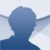# MalMath Android

6.0.18

## Are you struggling with math or can't find the solution to a math problem? The MalMath app solves any mathematical operations in a matter of seconds

Whether math makes you mad or you are mad about math, or if you simply need help solving a mathematical operation, this app is the solution to all your (math) problems. All you need to do is download the APK file, type the math problem, and get the solution step by step.

### Solutions to mathematical problems explained step by step

MalMath is an educational app that automatically solves math problems, equations, and operations with step-by-step directions. And that is only one of its functions.

To find the solution to a math problem, enter the operation in the calculator you will find on the home screen. And if you switch to premium, you can even take a photo to upload your math problem.

The main advantage this app has to offer is that it shows the result and a step to step explanation so you can learn to solve the equation yourself. You also have the option to see a graph.

Solve math problems step-by-step.

And that's not all: this tool also has an automatic math problem generator to help you study and practice. In this way, you can automatically create algebra, trigonometry, limits, differential calculus, and integrals problems of three levels of difficulty (easy, medium, and advanced).

In short, whether you need help with your math homework or you are an expert mathematician, this application will be extremely useful and practical. And to top it all, it is totally free.